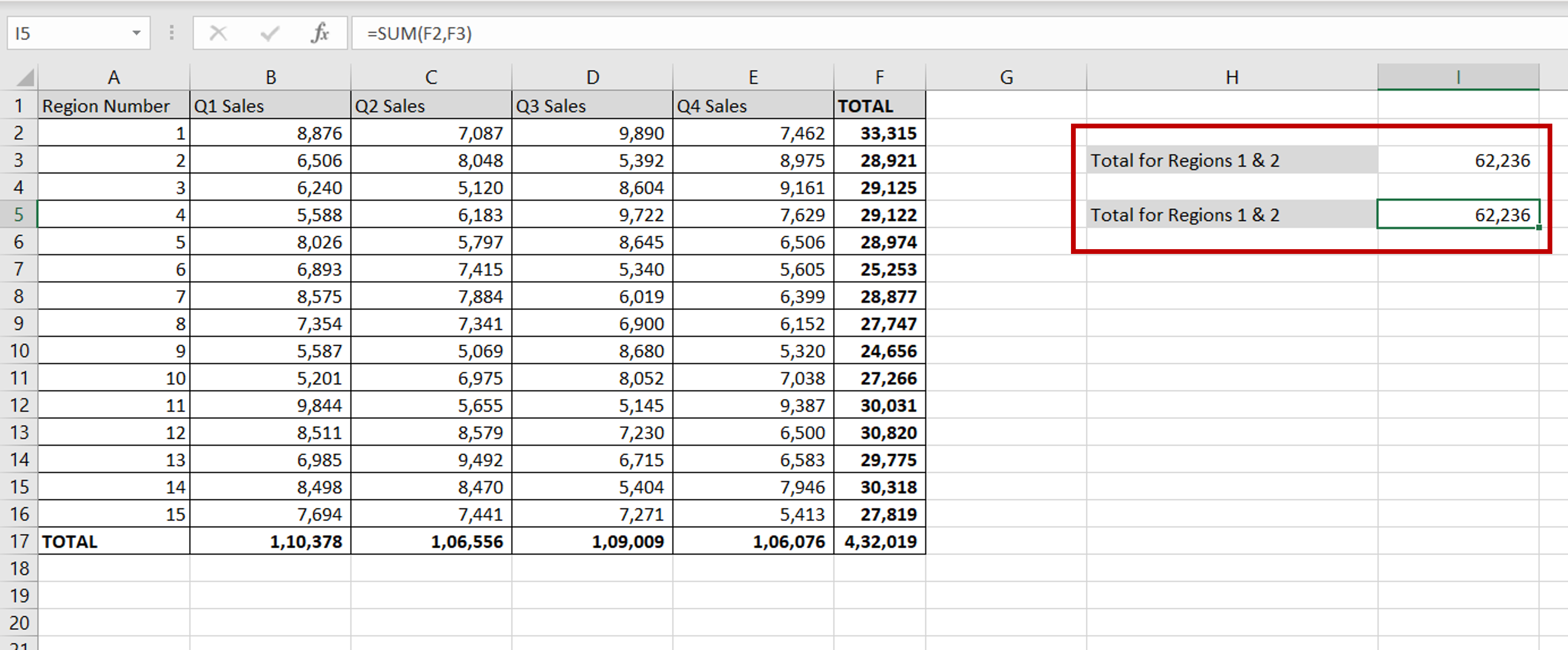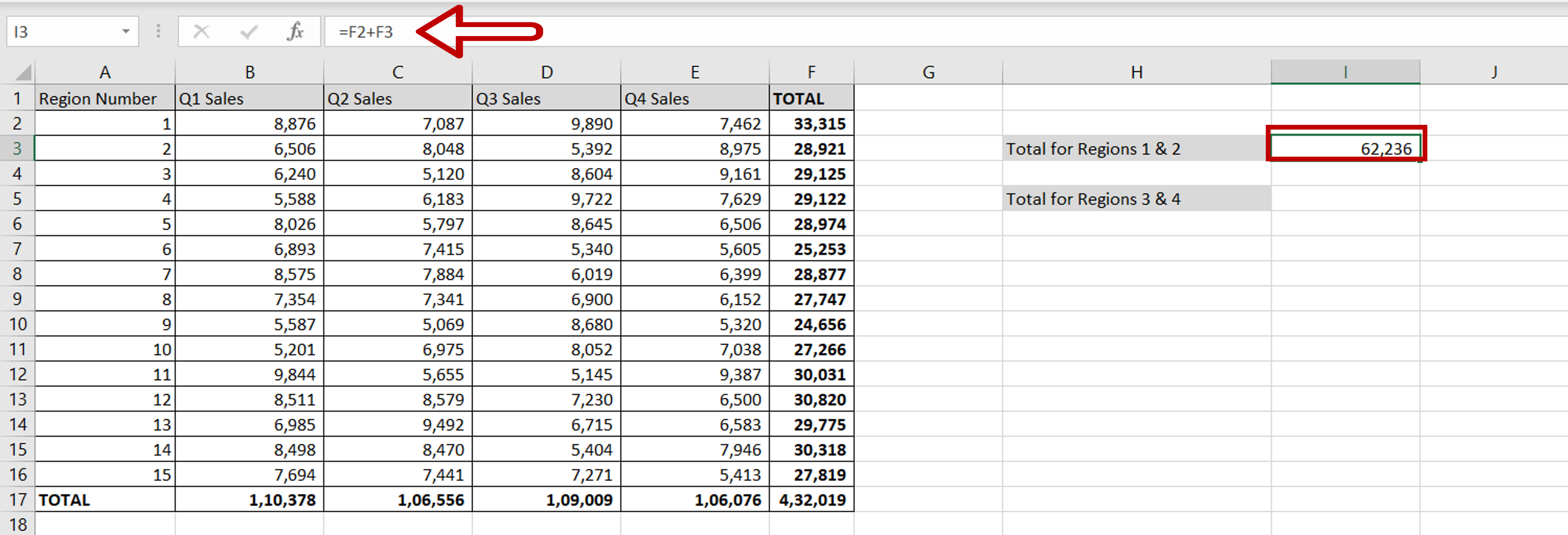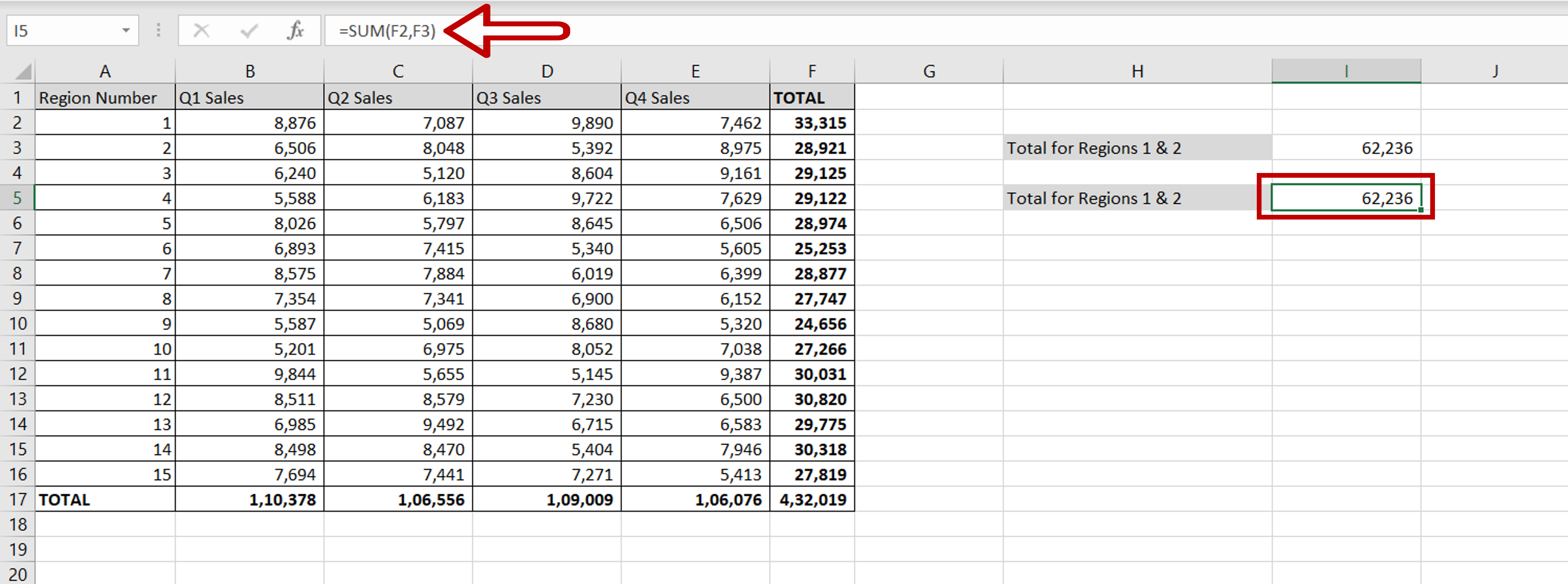# How to add two cells in Excel

You can watch a video tutorial here.Excel is widely used for calculations due to the several arithmetic operators and functions that it has. Instead of typing in numbers for calculations, you can use the cell references i.e. the address (Column header & Row number) of the cell in the calculation. When adding two cells in Excel, you can do so using the cell reference.

### Option 1 – Use the addition operator (+)– In the destination cell, type the formula using cell references:
= (Total for Region 1 + Total for Region 2)

– Press Enter

### Option 2 – Use the SUM() function– In the destination cell, type the formula using cell references:
= SUM(Total for Region 1,Total for Region 2)
– Press Enter

### Result – Compare the methods– Both options yield the same result